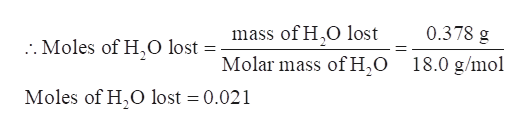# How many moles of water were lost if the amount of water lost was 0.378 grams?  Do not include units and assume three significant figures in all numbers.  Be sure to include the zero before the decimal if the number is less than one.

Question
5 views

How many moles of water were lost if the amount of water lost was 0.378 grams?  Do not include units and assume three significant figures in all numbers.  Be sure to include the zero before the decimal if the number is less than one.

check_circle

Step 1

The moles of water that were lost if the amount of water lost was 0.378 grams are to be calculated.

Step 2

The number of moles of a substance is defined as the mass of that substance divided by its molar mass.

Step 3

Given mass of water lost = 0.378 g

Mola...help_outlineImage Transcriptionclose.Moles of H,O lost = mass of H.O lost Molar mass of H,O 0.378 g 18.0 g/mol Moles of H,O lost 0.021 fullscreen

### Want to see the full answer?

See Solution

#### Want to see this answer and more?

Solutions are written by subject experts who are available 24/7. Questions are typically answered within 1 hour.*

See Solution
*Response times may vary by subject and question.
Tagged in

### General Chemistry